# 1st PUC Computer Science Previous Year Question Paper March 2014 (South)

Students can Download 1st PUC Computer Science Previous Year Question Paper March 2014 (South), Karnataka 1st PUC Computer Science Model Question Papers with Answers helps you to revise the complete Karnataka State Board Syllabus and score more marks in your examinations.

## Karnataka 1st PUC Computer Science Previous Year Question Paper March 2014 (South)

Time: 3:15 Hours
Max. Marks: 100

PART – A

I. Answer all the following questions. Each question carries one mark. (10 × 1 = 10)

Question 1.
Who invented Pascaline?
A French philosopher Blaise Pascal invented “pascaline” the first mechanical calculator.

Question 2.
Expand OCR.

Question 3.
Define coding.
The process of algorithmic solution or flowchart solution into a set of instructions in a programming language is called as coding.Question 4.
What is the difference between = and == operators?
The = is an assignment operator and == is a relational operator.

Question 5.
What is the purpose of end1?
The purpose of end1 is to break the line in output statement.

Question 6.
What is a compound statement?
A compound statement is a grouping of statements enclosed between the pair of flower braces ({}) in which each individual statement ends with a semi-colon.

Question 7.
What is the data type of an array subscript?
The data type of array subscript is integer or int.Question 8.
What are global variables?
A variable that is declared outside any function is known as a global variable.

Question 9.
A header in word processor is a special area at the top of every page, normally allocated in the top margin area.

Question 10.
What is the extension with which a work book is saved?
The extension file name with which a work book is saved is *.XLS.

PART – B

II. Answer any Five of the following questions. Each question carries two marks: (5 × 2 = 10)

Question 11.
Write the important features of second generation computers.
The two important features of the second generation computers are.

1. Use of symbolic or assembly language
2. Introduction of early versions of COBOL and FORTRAN high level languages

Question 12.
Name any two characteristics of impact printers.
The two characteristics of impact printers are

1. Print quality is low in some types
2. Uses ink ribbon to print
3. Less expensiveQuestion 13.
Define object code and source code.
1. Object code:
The object code is usually a machine code which can be understood directly by a specific type of CPU.

2. Source code:
The source code is any collection of computer instructions written using some human-readable computer language.

Question 14.
Write the syntax and example for WHILE loop.
Syntax of while loop: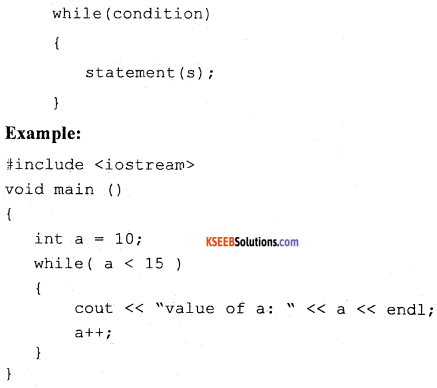Question 15.
Mention any two advantages of OOP.
Some of the advantages of object oriented programming are as follows: –

1. A clear modular structure can be obtained which can be used as a prototype and it will not reveal the details of the design.
2. Ease of maintenance and modification to the existing objects can be done with ease.
3. A good framework is provided which facilitates in creating rich GUI applications.Question 16.
What is type casting? Give one example.
Typecasting is making a variable of one type, such as an int, act like another type, a char, for one single operation.
Example:
void main ()
{
int a=5, b=2;
float c;
c = (float) a / b;
}

Question 17.
Mention any four string functions.
The four string functions are strupr(), strlwr(), strcpy() and strrev().

Question 18.
What is a mail merge? Write the steps for mail merge.
The mail merge helps to generate form letters by combining content of one document with content of address. In a situation where a letter is to be sent to address of many persons then mail merge is used.
The steps for mail merge is as follows;

• Step 1. Create a main document and type the letter.
• Step 2. Select data source document.
• Step 3. Insert merge field in the main document.
• Step 4. Select Finish and merge button.

PART-C

III. Answer any Five of the following Question. Each question carries three marks: (5 × 3 = 15)

Question 19.
Explain impact printers. Give an example.
Impact printers forms characters or images by striking a mechanism such as a print hammer or wheel against an inked ribbon, leaving an image on paper. For example, dot matrix printer.
Explain the different types of ROM.

1. PROM:
PROM stands for Programmable Read Only Memory and is initially blank. The manufacturer can write data/program on it by using special devices. However, once the program or data is written in PROM chip, it cannot be changed.

2. EPROM:
EPROM stands for Erasable Programmable Read Only Memory and is also initially blank. Unlike PROM, the data written in EPROM chip can be erased by using special devices and ultraviolet rays.

3. EEPROM:
EEPROM stands for Electrically Erasable Programmable Read Only Memory. This kind of ROM can be written or changed with the help of electrical devices. So data stored in this type of ROM chip can be easily modified.Question 20.
Write a note on ASCII code.

• ASCII (American Standard Code for Information Interchange) is the most widely used coding system to represent data.
• ASCII is used on many personal computers and minicomputers.
• ASCII is a 7-bit code that permits 2<sup>7</sup> = 128 distinct characters. The 128 different combinations that can be represented in 7 bits are plenty to allow for all the letters, numbers and special symbols.
• An eight bit was added. This allowed extra 128 characters to be represented. The extra 128 combinations are used for symbols such as Ç, ü, è, ©, ®, Æ, etc.

Question 21.
Write any three features of windows operating system.
General Features of windows operating system are

1. Fast start up and shutdown
2. Plug and play support for hardware devices
3. Supports all major networking protocols

Question 22.
What is an algorithm? Name any two characteristics of an algorithm.
An algorithm is defined as “step by step procedure to solve a given problem infinite number of steps”.

Characteristics of algorithm:

1. Every step should perform a single task
2. There should not be any confusion at any stage.
3. It must involve a finite number of steps.

Question 23.
What are pre-increment and post-increment operators? Give examples.
The pre-increment operator is a unary operator and is used to increment the value of variable before using in the expression i.e. value is first incremented and then used in the expression.

Example:
Y = 5;
cout<< ++Y;
output: 6.
The post-increment operators is also unary operator and is used to increment the value of variable after the use of expression.
For example;
Y=5;
cout<< Y++;
output: 5.Question 24.
Briefly explain the input and output operators in C++ with their syntax and example.
1. Input Operator:
Syntax:
cin >> variable.
Example:
The statement cin>> num;
is an input statement and causes the program to wait for the user to type in a number. It takes the value from the keyboard and assigns it to the variable on its right.

2. Output Operator:
Syntax: cout<< expression or manipulator
Example:
The statement Cout<< “ the numbers”;
It is an output statement and causes the program to print the message on the standard output.

Question 25.
Define two-dimensional array. Write its declaration syntax and give one example.
Two dimensional array is a collection of elements of similar type that share a common name, structured in two dimensions. Each element is accessed by two index values.
Syntax:
<data-type> <array-name> [<size1>] [<size2>];
Example:
int a [ 10] [ 10];

Question 26.
What is a structure? Write the syntax for structure definition.
A structure is a collection of variable which can be same or different types.
Syntax for structure definition:PART – D

IV. Answer any seven of the following questions. Each question carries Five marks: (7 × 5 = 35)

Question 27.
Explain the characteristics of computers in detail.
The characteristics of computers are:
1. Speed:
Computers are fast in doing calculations. The speed of the computer is measured in terms of million instruction per second (MIPS).

2. Storage Capacity:
Computers come with large amount of memory. They can hold lot of data. Computers can show a particular piece of information from large amount of data in a short time.

3. Diligence:
After doing work for sometime, humans become tired but computers do not become tired. They work continuously. In fact, some computers which control telephone exchanges are never stopped. This is called diligence.

4. Accuracy:
The results that the computers produce are accurate provided data and programs are reliable.

5. Versatality:
We can use computer to perform completely different type of work at the same time.

6. Reliability:
Computers work for many years without any problem. Few computers in the world are never switched off.Question 28.
1. Perform 14(10) + 18(10) using binary additions.
The 14(10) = 1110(2) and 18(10) = 10010(10).2. Subtract (10) from 15(10) using 2’s complement method.
1 1 1 1 – minuend
0 1 1 1 – subtrahend

The subtrahend 0 1 1 1 one’s complement is 1 0 0 0
Two’s complement =Now add this to minuend i.e.,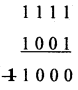Answer is: 1 0 0 0(2)

Question 29.
Explain the various types of errors detected during testing.
The different program errors are as follows;
1. Synta’x error:
It occurs when there happens to be a misuse of a programming language’s instructions. It happens at the time of compilation. Such errors need to be rectified before proceeding further.

2. Semantic errors:
An error, which occurs due to the incorrect logic and a solution called semantic error. It also occurs due to wrong use of grammar in program.

Runtime Error occurs at run-time. Such error cause a program to end abruptly or even cause system shut-down. Such errors are hard to detect.

3. Logical Error:
It may happen that a program contains no syntax or run-time errors but still it doesn’t produce the correct output. It is because the developer has not understood the problem statement properly. These errors are hard to detect as well.

Question 30.
What are relational operators? Explain any four relation operators in C++.
The operators which perform operation of relation between two operands called relational operators.
The > (greater than) operator: if (10 > 5)? Output: True. If ( 5 > 10)? Output: False
The < (lesser than) operator: if (15 < 25)? Output: True. If ( 56 < 26)? Output: False
The >= (greater than or equal to) : if (10 >= 10)? Output: True. If (5 >= 10)? Output: False
The <= (Lesser than or equal to): if (6 <= 10)? Output: True. If ( 16 <= 10)? Output: FalseQuestion 31.
Explain if-else-if statement with general syntax and give one example.
The if else if statement is an extension of the “if else” conditional branching statement. When the expression in the “if’ condition is “false” another “if else” construct is used to execute a set statements based on expression.
Syntax: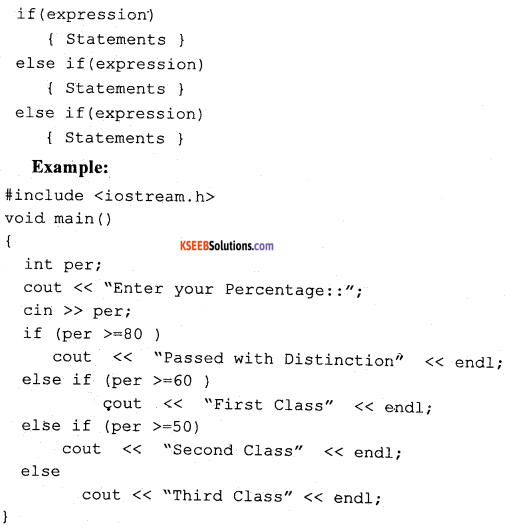Result:
Enter your Percentage : : 60
First Class

Question 32.
Draw a flowchart to find the factorial of a number.Question 33.
Write a C++ program to find the sum and average of n numbers.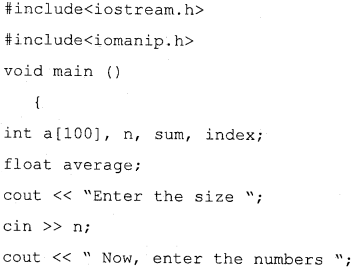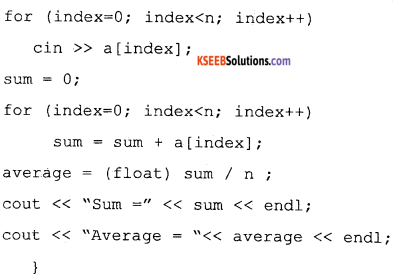Question 34.
Explain the working of functions with arguments and no return values.
The calling function main() gives the function call to the called function by passing arguments or values. Then the called function takes the values and performs the calculations and gives the output in itself but no value will be sent back to the calling function.
For example,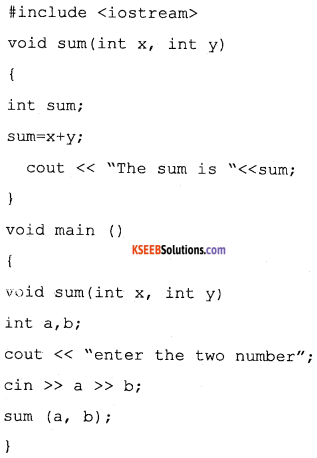In the above example, sum() is a user defined function. main() is a calling function gives a function call sum (a, b); with actual arguments a, b. The copy of values of a and b are sent to formal arguments x and y in the called function stim().

The function sum() perform addition and gives the result on the screen by itself. Here no value is sent back to calling function main() can be observed. These kinds of functions are called “Function with argument but no return values”.Question 35.
What is a worksheet? Explain the steps used to change the size of rows and columns in a worksheet.
An Electronic spreadsheet is application software and worksheet is divided into rows and columns to store items like student marks, stocks, income and expenses, debits and credits.

The steps used to change the row size and columns width in a work sheet.
Select cell → format → row height → height in numbers
Select cell →format → column height → height in numbers

Question 36.
Explain the various options of the formatting toolbar in the spreadsheet.
It is a toolbar that has the icons that provide the various formatting settings for cells, columns, rows and data in Excel. For example, it can allow to change the colours of rows, cells and columns. It allows to change fonts. It allows to set the alignment for data in cells. It allows to apply borders to cells.

It allows to change the format of numbers, like adjusting the amount of decimals they have or changing them to percentage format. All of these and other things can be done with it.

1. Format Cells Dialog Box:
For a complete list of formatting options, right-click on the highlighted cells and choose Format Cells from the shortcut menu or select Format > Cells from the menu bar.

2. Number tab:
The data type can be selected from the options on this tab. Select General if the cell contains text and numbers, or a different numerical category if the cell is a number that will be included in functions or formulas. The number tab can be used to limit the number decimal places,

3. Alignment tab:
These options allow you to change the position and alignment of the data within the cell. For example, right, left, center, vertical alignments, text wrap, etc.,

4. Font tab:
All of the font attributes are displayed in this tab including font face, size, style, and effects. For example, bold, italicize, underline, increase/decrease the font size, changing the color of font, fill, etc.,

5. Border and Pattern tabs:
These tabs allow us to add borders, shading, and background colors to a cell.

Question 37.
Explain the various benefits of email.